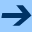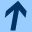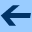StringBuilder

# StringBuilder

We often want to build up a large string from small pieces. As an example, consider the method joinToString of lists and sets. Let's implement a simple version for lists (join1.kts):

fun join(l: List<Int>): String {
var s = ""
for (e in l) {
if (s.isEmpty())
s = e.toString()
else
s = s + ", " + e.toString()
}
return s
}

This works, as can be seen from this test:
>>> :load join1.kts
>>> val s = (1..10).toList()
>>> join(s)
1, 2, 3, 4, 5, 6, 7, 8, 9, 10


However, remember that string objects are immutable. When adding another number to the current string s, a completely new string has to be created, and all the characters are copied from the old string to the new one. When the length of the list is large, this could be a slow operation. Let's write some code to measure the running time of our function join (join2.kts):

import java.lang.System.currentTimeMillis

fun join(l: List<Int>): String {
var s = ""
for (e in l) {
if (s.isEmpty())
s = e.toString()
else
s = s + ", " + e.toString()
}
return s
}

val n = args.toInt()
val a = (1 .. n).toList()

val t0 = currentTimeMillis()
val s = join(a)
val t1 = currentTimeMillis()

println("Creating a string with ${a.size} integers took${t1 - t0} milliseconds")


The output is

$kts join2.kts 100 Creating a string with 100 integers took 1 milliseconds$ kts join2.kts 1000
Creating a string with 1000 integers took 3 milliseconds
$kts join2.kts 10000 Creating a string with 10000 integers took 529 milliseconds$ kts join2.kts 20000
Creating a string with 20000 integers took 1728 milliseconds
$kts join2.kts 40000 Creating a string with 40000 integers took 6680 milliseconds$ kts join2.kts 80000
Creating a string with 80000 integers took 27939 milliseconds


Note how the code gets slower and slower: Doubling the number of elements causes the runtime to increase by a factor of roughly four.

To solve this problem, we need a mutable string type: an object where we can add more characters at the end without copying everything. The Java library provides the data type StringBuilder which is essentially a mutable String. Once the string has been fully built, we can convert it to a normal string by calling its toString() method.

Here is the new join function (join3.kts):

fun join(l: List<Int>): String {
var s = StringBuilder()
for (e in l) {
if (s.isEmpty())
s.append(e.toString())
else {
s.append(", ")
s.append(e.toString())
}
}
return s.toString()
}


And this is much faster:

$kts join3.kts 80000 Creating a string with 80000 integers took 35 milliseconds$ kts join3.kts 1000000
Creating a string with 1000000 integers took 113 milliseconds


Note that even a million numbers are no problem now.

StringBuilder objects support most string methods, and have some other methods that turn them into mutable strings:

• s.append(ch) appends the character ch;
• s.append(t) appends the string t;
• s.append(n) appends the string representation of the number n (which can be any number type such as Int, Double, Long, etc.);
• s.append(x) appends the string representation of any object (by calling their toString() method);
• s.delete(i, j) deletes characters from index i (inclusive) until j (exclusive);
• s.insert(i, t) inserts the string t at index i;
• s.toString() returns the contents as a normal, immutable string.StringBuilder